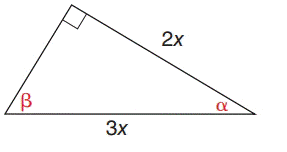Chapter 11.1, Problem 26E### Elementary Geometry for College St...

6th Edition
Daniel C. Alexander + 1 other
ISBN: 9781285195698

#### Solutions

Chapter
Section### Elementary Geometry for College St...

6th Edition
Daniel C. Alexander + 1 other
ISBN: 9781285195698
Textbook Problem
1 views

# In Exercises 21 to 26, find the measures of the angles named to the nearest degree.To determine

To measure:

The angle named to the nearest degree.

Explanation

In a right angle triangle,

1) The side PQ, which is opposite to the right angle PRQ is called the hypotenuse.

(The hypotenuse is the longest side of the right triangle.)

2) The side RQ is called the adjacent side of angle θ.

3) The side PR is called the opposite side of angle θ.

Formula used:

sinθ=oppositehypotenuse

Given:

By using Pythagoras theorem, that is

c2=a2+b2

Let b be the adjacent side of the triangle.

From, the diagram we have c=3x and a=2x

(3x)2=(2x)2+b29x2=4x2+b2b2=9x24x2b2=5x2b=5x2b=2.24x

For angle α, the opposite side is 2x and hypotenuse side is 3x

### Still sussing out bartleby?

Check out a sample textbook solution.

See a sample solution

#### The Solution to Your Study Problems

Bartleby provides explanations to thousands of textbook problems written by our experts, many with advanced degrees!

Get Started

#### In Exercises 914, evaluate the expression. 14. 3543183

Applied Calculus for the Managerial, Life, and Social Sciences: A Brief Approach

#### Prove Equation 4.

Single Variable Calculus: Early Transcendentals, Volume I

#### Using for |x| < 1,

Study Guide for Stewart's Multivariable Calculus, 8th

#### The average value of f(x) = 3x2 + 1 on the interval [2, 4] is: a) 29 b) 66 c) 58 d) 36

Study Guide for Stewart's Single Variable Calculus: Early Transcendentals, 8th